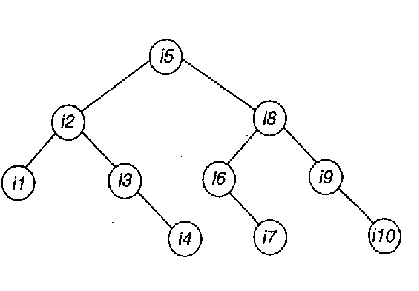# Data Structures - Sorting & Searching

26:

The number of swappings needed to sort the numbers 8, 22, 7, 9, 31, 19, 5, 13 in ascending order, using bubble sort is

 A. 11 B. 12 C. 13 D. 14 Answer Report Discuss Option: D Explanation : Click on Discuss to view users comments. pavittar said: (9:18pm on Monday 13th May 2013) 13 answer correcrt plz tell how 14 swappings Vijay Singh said: (9:12pm on Wednesday 24th July 2013) Is there any formula for this? plz comment. Write your comments here:
27:

The average successful search time taken by binary search on a sorted array of 10 items is

 A. 2.6 B. 2.7 C. 2.8 D. 2.9 Answer Report Discuss Option: D Explanation : The 10 items i1, i2, ..., i10 may be arranged in a binary search trees as in Fig   To get i5, the number of comparision needed is 1; for i2, it is 2; for i8 it is 2; for i1 it is 3, and so on. The average is (1+(2+2) +(3+3+3+3)+(4+4+4))/10, i.e., 2.9.Click on Discuss to view users comments. Write your comments here:
28:

The average successful search time for sequential search on 'n' items is

 A. n/2 B. (n-1)/2 C. (n+1)/2 D. log (n)+1 Answer Report Discuss Option: C Explanation : If the search key matches the very first item, with one ocmparision we can terminate. If it is second, two comparisons , etc. So, average is (1+2+...+n)/n .i.e. (n+1)/2 Click on Discuss to view users comments. Write your comments here:
29:

The running time of an algorithm T(n), where 'n' is the input size, is given by

T(n) = 8T(n/2) + qn, if n>1

Where p,q are constants. The order of this algorithm is

 A. n2 B. nn C. n3 D. n Answer Report Discuss Option: C Explanation : Click on Discuss to view users comments. Write your comments here:
30:

L:et m, n be positive integers. Define Q (m, n) as

Q (m, n) = 0, if m>n

Then Q (m, 3) is (a div b, gives the quotient when a is divided by b)

 A. a constant B. p x (m mod 3) C. p x (m div 3) D. 3 x p Answer Report Discuss Option: C Explanation : Let m>n. Let m/n yield a quotient x and remainder y. So, m= n*x+y and y